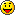Solution to Lab 10′s Probability Challenge

This is the solution to Lab 10. II.3. A few of you asked for it, so here it is!

For the first part, we first calculate the probability of at least one person picking his/her hat back up. We’ll let$E_i$ be the event that the ith person picking up his/her hat. We can bound the probability by just taking the sum of the probability that each person takes his/her own hat,$\displaystyle\sum_{i=1}^N P(E_i)$. However, this is overestimating by the sum of the probabilities that two people select their own hats, so we need to subtract out the term$\displaystyle\sum_{i_1 < i_2} P(E_{i_1}E_{i_2})$. But now, we’re underestimating by the sum of the probabilities that three people got their hats. This flips back and forth, adding, and subtracting terms. There’s actually a rule for this: inclusion-exclusion, but as usual, it’s better to just know the concept. So the probability of any of them selecting a hat is$P(E_1 \cup E_2 \cup E_3 \cup \cdots) = \displaystyle\sum_{i=1}^N P(E_i) - \displaystyle\sum_{i_1 < i_2} P(E_{i_1} E_{i_2}) + \cdots + (-1)^{n+1} \displaystyle\sum_{i_1 < i_2\cdots

If we think of this problem as a list of N numbers, where the ith number is the number of the hat drawn by the ith person, then there are N! possible outcomes. Furthermore, the event that each of n people selectes his/her own hat can occur in any of$(N-n)(N-n-1)\cdots 3 \cdot 2 \cdot 1 = (N-n)!$ possible ways. For the remaining N-n men, the first can select any of N-n hats, the second can then select any of N-n-1 hats, and so on. Hence we see that$P(E_{i_1} E_{i_2} \cdots E_{i_n}) = \frac{(N-n)!}{N!}$.

Also, as there are${N \choose n}$ terms in$\displaystyle\sum_{i_1 < i_2\cdots , we can see that$\displaystyle\sum_{i_1 < i_2\cdots

and, so we can combine everything, to get that$1-P(E_1 \cup E_2 \cup E_3 \cup \cdots) = 1- (1- \frac{1}{2!} + \frac{1}{3!} - \cdots + (-1)^{N+1} \frac{1}{N!})$.

For the second part, we apply what we learned in the first. The probability that k people can select their own hats is equal to the number of ways in which every other N – k person does not pick his/her own hat. We know the probability that none of the N – k people picking their own hats is just$1-P(E_1 \cup E_2 \cup E_3 \cup \cdots) = 1- 1+ \frac{1}{2!} - \frac{1}{3!} + \cdots + \frac{(-1)^{N-k}}{N-k!}$.

Hence, the number of ways that the people each picking their own hats happens to be the k people we’re looking for is$(N-k)!\left[1- 1+ \frac{1}{2!} - \frac{1}{3!} + \cdots + \frac{(-1)^{N-k}}{N-k!}\right]$

Furthermore, there are${N \choose k}$ ways to pick k people from N people, so there are$(N-k)!\left[1- 1+ \frac{1}{2!} - \frac{1}{3!} + \cdots + \frac{(-1)^{N-k}}{N-k!}\right]$
ways that k people pick their own hats.

So the probability we want is just$\displaystyle\frac{(N-k)!\left[1- 1+ \frac{1}{2!} - \frac{1}{3!} + \cdots + \frac{(-1)^{N-k}}{N-k!}\right]}{N!}$

which simplifies to$\displaystyle\frac{1- 1+ \frac{1}{2!} - \frac{1}{3!} + \cdots + \frac{(-1)^{N-k}}{N-k!}}{k!}$

Let me know if you got something similar!

One response to “Solution to Lab 10′s Probability Challenge”

1.I spent a LOT of time on this, and got the idea, but after 2 and a half hours, the math got muddled up so I messed up the plus signs and minus signsStill, I did understand this, and then of course, I remembered my friend had asked me this question before, so I googled ” Derangement “, and I found it!
So after going through multiple proofs on the internet, I saw how the first part can be generalized to 1/e, which is just kinda nifty.
“e” pops up yet again!